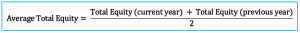# Average Total Equity

## Definition

Average total equity is the average carrying value of equity that are recorded on the balance sheet at the different reporting dates. Usually, the carrying value of equity at the end of the previous year and those at the end of the current year are used in the calculation to find average total equity on the balance sheet.

We usually use the average total equity to calculate the return on equity or ROE as it is one of the main financial ratios to measure the company’s ability in the utilization of the resources, its equity, to generate the profit.

## Average Total Equity Formula

We can calculate average total equity by using formula of total equity value at the end of the current year plus total equity value at the end of the previous year and then divide the result by two.## Average Total Equity Example

For example, we have the extracted balance sheet as below:

Extracted Balance Sheet 31 Dec 2019 31 Dec 2018
Current liabilities \$ \$
Interest payables 600 788
Account payables 4,600 3,800
Accruals 2,800 2,650
Other current liabilities 1,200 800
9,200 8,038
Non-current liabilities
Note payables 35,000 45,000
Other non-current liabilities 3,155 3,155
38,155 48,155
Total liabilities 47,355 56,193
Equity
Share capital 65,000 65,000
Retained earnings 29,572 17,500
Profit/(Loss) current year 15,360 12,072
Total equity 109,932 94,572
Total liabilities and equity 157,287 150,765

Calculate average total equity and ROE in 2019 based on the extracted balance sheet above.

## Calculate Average Total Equity

With the average total equity formula above, we can calculate as below:

Total equity value at 31 Dec 2019 = \$109,932

Total equity value at 31 Dec 2018 = \$94,572

Then

Average Total Equity = (109,932+94,572) / 2 = \$102,252

So, the average total equity is \$102,252 which we can use to calculate the return on equity ratio.

In this case, we can calculate return on equity by using the net profit in 2019 and the average return on equity figure as below:

Return on Equity = 15,360 / 102,252 = 15.02%